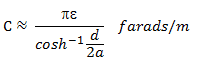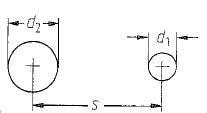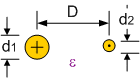# How do I calculate the capacitance of two unequal lines?

Tae Hwan Kim
The capacitance formula for equal line is as follows. :* my question :
I want to know the capacitance formula for unequal lines and its solving process .Thanks in advance for any hint.

#### Attachments

the_emi_guy
There probably isn't a formula for this. Even the formula for equal lines is an approximation.

You can use a field solver, such as FastCap, to determine the capacitance per length of your structure.

Mentor
If the distance between the wires is much larger than the wire diameters, I would expect the inverse average of the inverse values to give a reasonable approximation. You can imagine a conducting plate in the middle. Calculate the capacitance for both sides with the formula in post 1 (multiplied by 2 because we have only one side) and then treat your system as two capacitors in series.

•the_emi_guy
Gold Member
For any RF guru around, there is a formula for the impedance of two parallel, unequal conductors; perhaps this will give a clue for unit capacitance.
The below from: http://www.rfcafe.com/references/electrical/transmission-lines.htm (I have a text with the same formula, this was easier to copy!)

Two Conductors in Parallel (Balanced)
of Unequal Diameters

Z0= (60/ε½) cosh-1 (N)
Z0 = (60/ε½) cosh-1 (N)
/EDIT:

N = ½[(4D2/d1d2) - (d1/d2) - (d2/d1)]#### Attachments

Last edited:
•mfb
Gold Member
If the distance between the wires is much larger than the wire diameters, I would expect the inverse average of the inverse values to give a reasonable approximation. You can imagine a conducting plate in the middle. Calculate the capacitance for both sides with the formula in post 1 (multiplied by 2 because we have only one side) and then treat your system as two capacitors in series.
I don't think the equipotential plane will be quite in the middle.

Mentor
There is an infinite set of these planes, with the given approximation the middle should be close to an (!) equipotential plane.

If you plot the equipotential lines about two parallel filaments you will see that they are circular and all pass between the filaments, but they are not centred on the filaments. There is only one plane equipotential surface, midway between the filaments.

Given the radii and centre separation of two unequal cylindrical conductors, you can map the two conductor surfaces to be coincident with a circular equipotential surface about each filament.

When the conductors have different radii, the equipotential plane of symmetry between the filaments will no longer be centred between the conductor centres.

See:

The Capacitance of Two-Wire Transmission Lines by prof.Eric Chihming Tsai

http://moodle.ncku.edu.tw/mod/resource/view.php?id=620674

•mfb and Baluncore
•